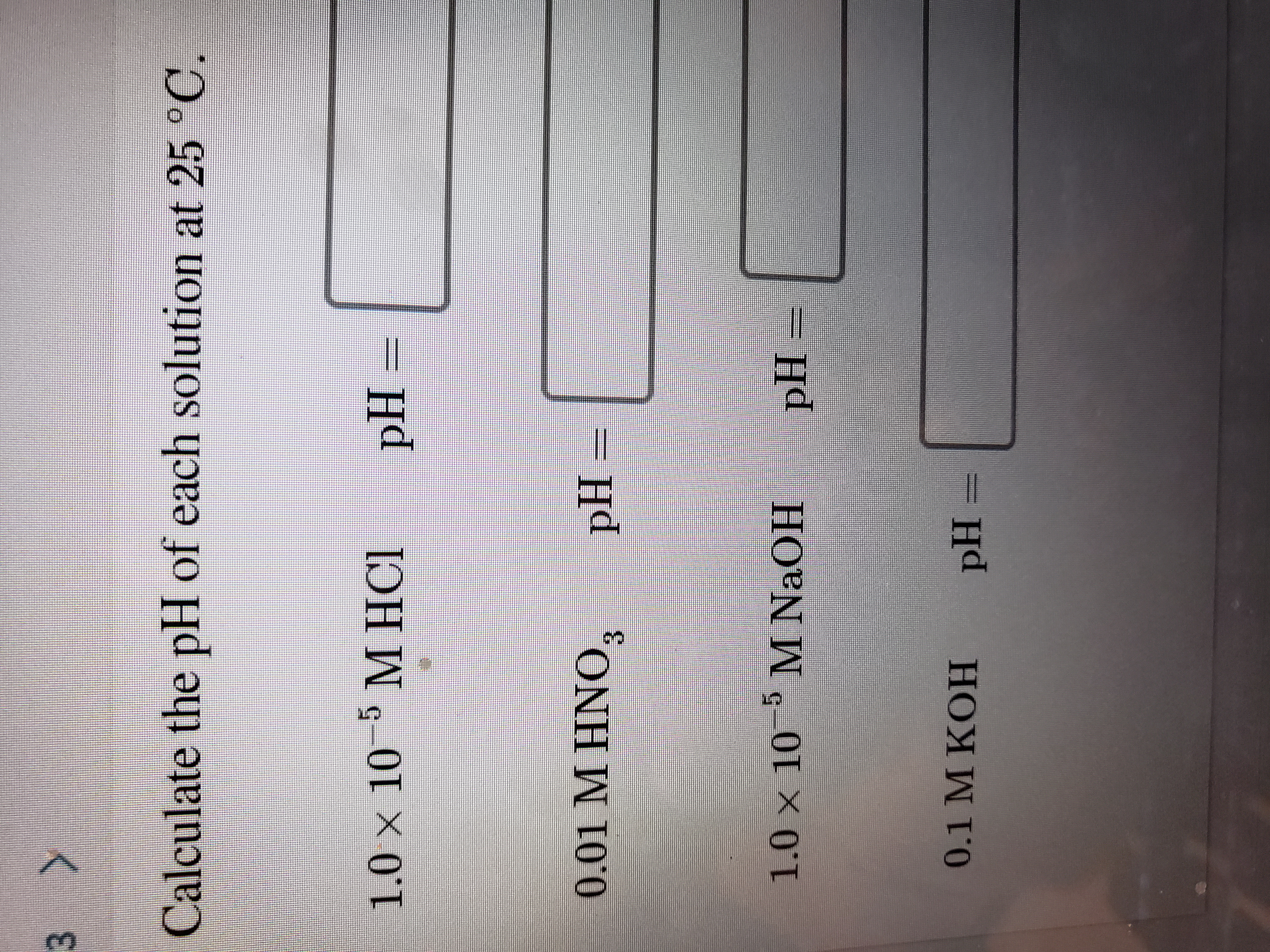# 3 >Calculate the pH of each solution at 25 °C.5 М НСI1.0 × 10M HCIpH =0.01 M HNO,pH=3.1.0 x 10 M NaOHpH0.1 M KOHpH =

Question
97 viewshelp_outlineImage Transcriptionclose3 > Calculate the pH of each solution at 25 °C. 5 М НСI 1.0 × 10 M HCI pH = 0.01 M HNO, pH= 3. 1.0 x 10 M NaOH pH 0.1 M KOH pH = fullscreen
check_circle

Step 1

Hello. Since your question has multiple sub-parts, we will solve first three sub-parts for you. If you want remaining sub-parts to be solved, then please resubmit the whole question and specify those sub-parts you want us to solve.

Step 2

The HCl, being strong electrolyte, completely dissociate in the solution and give the hydrogen ion. The concentration of H+ ion is equal to 1.0×10-5 M. The pH for 1.0×10-5 M HCl solution is calculated as shown below. The pH for 1.0×10-5 M HCl solution is 5.

Step 3

The HNO3, being strong electrolyte, completely dissociate in the solution and give the hydrogen ion. The concentration of H+ ion is equal to 0.01 ...

### Want to see the full answer?

See Solution

#### Want to see this answer and more?

Solutions are written by subject experts who are available 24/7. Questions are typically answered within 1 hour.*

See Solution
*Response times may vary by subject and question.
Tagged in
ScienceChemistry

### Equilibrium Concepts